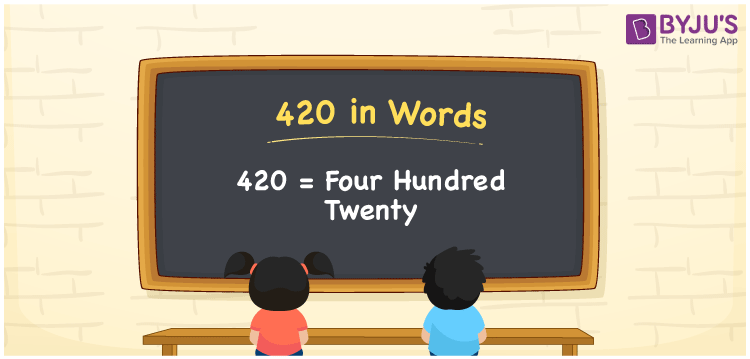# 420 in Words

420 in words is written as Four hundred twenty. Generally, we use the word form of numerals to spell or express a certain quantity or measurement. For example, the circular field area is given as 420 square meters; we can say that “The area of this circular field is Four hundred twenty square meters”. In this article, you will learn how to convert the number 420 into words, along with some interesting facts about 420.

 420 in words Four hundred twenty Four hundred twenty in Numbers 420

## 420 in English Words

We generally use the English alphabet to write numbers in words. Thus, we spell 420 in English words as “Four hundred twenty”.## How to Write 420 in Words?

To write 420 in words, we need to derive the place values for all the digits, i.e. for 4, 2, and 0. This can be done as follows.

 Hundreds Tens Ones 4 2 0

Here, ones = 0, tens = 2, hundreds = 4

These digits can be expanded according to their place values as follows.

4 × Hundred + 2 × Ten + 0 × One

= 4 × 100 + 2 × 10

= 400 + 20

= Four hundred + twenty

= Four hundred twenty

Therefore, 420 in words = Four hundred twenty

We know that 420 is a natural number that precedes 421 and succeeds 419.

420 in words – Four hundred twenty

Is 420 an even number? – Yes

Is 420 an odd number? – No

Is 420 a prime number? – No

Is 420 a composite number? – Yes

Is 420 a perfect square number? – No

Is 420 a perfect cube number? – No

## Frequently Asked Questions on 420 in Words

### How do you say 420 in English words?

We can spell the number 420 in English words as Four hundred twenty.

### Express the value 596 + 420 in words.

596 + 420 = 1016 Hence, the value of 596 + 420, i.e. 1016 in words is Thousand and sixteen.

### How to write Rs. 420 in words on a cheque?

On a cheque, we may write Rs. 420 in words as “Four hundred twenty rupees only”.